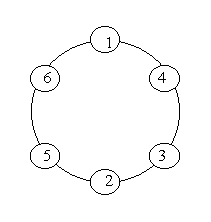19 May 2014

# 题目

#### `Problem Description`

```A ring is compose of n circles as shown in diagram. Put natural number 1, 2, ..., n into each circle separately, and the sum of numbers in two adjacent circles should be a prime.
Note: the number of first circle should always be 1.```

#### `Input`

``````n (0 < n < 20).
``````

#### `Output`

``````The output format is shown as sample below. Each row represents a series of circle numbers in the ring beginning from 1 clockwisely and anticlockwisely. The order of numbers must satisfy the above requirements. Print solutions in lexicographical order.

You are to write a program that completes above process.

Print a blank line after each case.
``````

#### `Sample Input`

``````6
8
``````

#### `Sample Output`

``````Case 1:
1 4 3 2 5 6
1 6 5 2 3 4

Case 2:
1 2 3 8 5 6 7 4
1 2 5 8 3 4 7 6
1 4 7 6 5 8 3 2
1 6 7 4 3 8 5 2
``````

# 解题思路

``````深度优先搜索，最难的也就是判断头尾两个相加起来是否为质数

``````